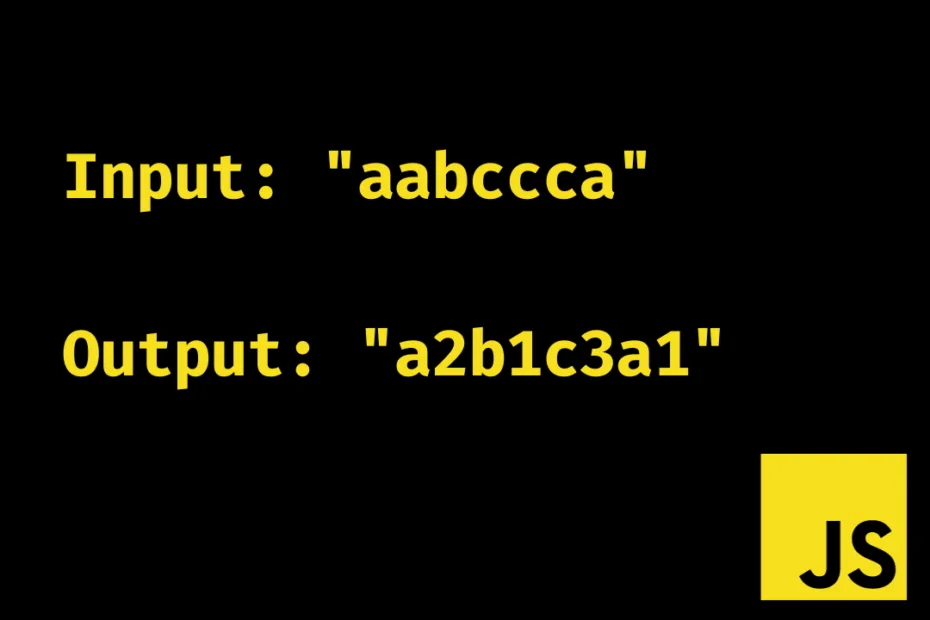# Consecutive characters count in String with JavaScript

Print the given string with the consecutive characters count next to each character, for example, Input: “aabccca” then Expected Output: “a2b1c3a1”. I tried a couple of things and decided to go with a simple for loop as this gives us access to the index with a single iteration, i.e., Linear time complexity O(n).

## Code to Count Consecutive characters

``````// JavaScript code to print Consecutive characters of a String

const getCount = (inputString = "") => {
let outputString = "";
let characterCount = 1;

for (let i = 0; i < inputString.length; i++) {
const character = string[i];
const nextCharacter = string[i + 1];

if (character === nextCharacter) {
characterCount++;
} else {
outputString += `\${character}\${characterCount}`;
characterCount = 1;
}
}

return outputString;
};

const string = "aabcca";
console.log(getCount(string));
// Output: a2b1c2a1``````

## Conclusion

The code is simple and straightforward but I am curious if there are more ways to do the same. One of my previous posts could be an interesting read as I have solved the same problem in four different methods – Count Vowels in a String with JavaScript.

Tags: